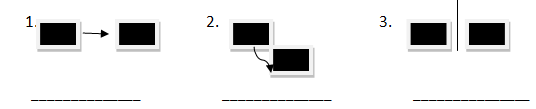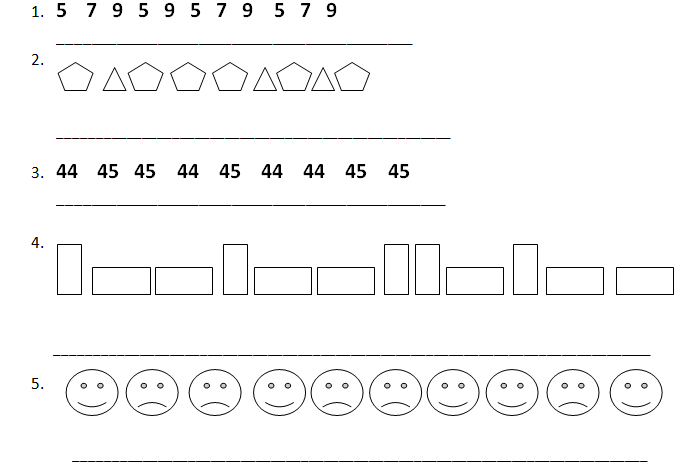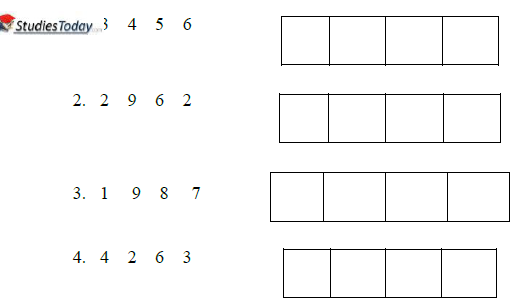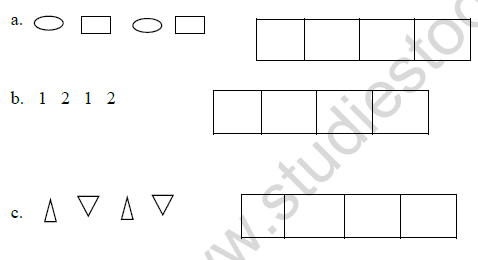# CBSE Class 1 Maths Revision Worksheet Set A

Download printable Mathematics Class 1 Worksheets in pdf format, CBSE Class 1 Maths Revision Worksheet has been prepared as per the latest syllabus and exam pattern issued by CBSE, NCERT and KVS. Also download free pdf Mathematics Class 1 Assignments and practice them daily to get better marks in tests and exams for Grade 1. Free chapter wise worksheets with answers have been designed by Standard 1 teachers as per latest examination pattern

## Mathematics Worksheet for Class 1

Class 1 Mathematics students should refer to the following printable worksheet in Pdf in Grade 1. This test paper with questions and solutions for Standard 1 Mathematics will be very useful for tests and exams and help you to score better marks

### Class 1 Mathematics Worksheet Pdf

Metric Measurement

Use centimeters to measure the lengths of short objects. E.g length of your finger.

Use meters to measure distances and the lengths of longer objects. E.g length of your bed room.

Use grams to measure the mass of light objects. E.g the mass of a spoon.

Use kilograms to measure the mass of heavier objects. E.g the bag of potatoes.

1. Draw a line of symmetry. The two parts will be congruent.2. Write the words turn, flip, or slide that names the move.Pattern Units

3. Read the pattern. Circle the mistake. Correct the pattern.Q2. Put > < or =

1. 2 ____ 4            6. 8 ____ 6

2. 6 ____ 7             7. 5____ 3

3. 10 ____ 1           8. 9 ____ 0

4. 6 ____ 1

5. 8____ 4

Q3. Ring all the numbers bigger than 10

a. 1      6     3     15     9       8

b. 17    8     5     20     13      3

c. 5     20    18     8     11     18

d. 0     15    7       4     3       29

Q4. Ring the numbers smaller than 10

a. 2         0     14       13      7

b. 19       9      9        20      22

c. 17       18     8       30       32

d. 15       0      20      3        13

Q 5. Arrange from biggest to smallest.Q 6. Complete the pattern.